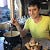Leetcode 2103: Rings and Rods

In this problem, we are given a list of rings going into rods, and we should return how many rods have rings with all the possible colors.

There are rings and each ring is either red, green, or blue. The rings are distributed across ten rods labeled from

Leetcode 2141: Maximum Running Time of N Computers

In this problem, we try to figure out how long we can run computers using a list of batteries. We have to use different batteries at any moment and switching them can be done any time (as long as it is a whole minute). …

Leetcode 2140: Solving Questions With Brainpower

In this problem, we are trying to find a testing strategy to optimize the number of points in a test giving the following rules:

You are given a 0-indexed 2D integer array where .

The array describes the questions of an exam, where you have to…

Leetcode 2139: Minimum Moves to Reach Target Score

In this exercise, we are trying to obtain a target number using and operations, we also have a limited number of operations:

You are playing a game with integers. You start with the integer and you want to reach the integer .
In one move, you can…

Leetcode 2138: Divide a String Into Groups of Size k

In this problem, we divide a String into a list of substrings of size k. In the last String is not of size k, we complete the string with a character until the string is of size k.

Example:
s = "abcdef", k = 4, fill = 'x'
Return…

Leetcode 2115: Find All Possible Recipes from Given Supplies

In this problem, we are trying to figure the list of recipes that we can make out of a list of ingredients in unlimited quantity. The difficulty comes from the fact that in the list of ingredients necessary for each recipe, there can be another recipe itself.

You have information…

Leetcode 2108: Find First Palindromic String in the Array

In this problem, we want to find the first String that is a palindrome in an array of Strings.

Given an array of strings , return the first palindromic string in the array. If there is no such string, return an empty string .

A string is palindromic if it…

Leetcode 2135: Count Words Obtained After Adding a Letter

In this Leetcode problem, we are asked to transform some words from an array of words to target words. Any word from the initial array can be used to be transformed in a target word using a specific transformation rule.

You are given two 0-indexed arrays of strings and…

Leetcode 2134: Minimum Swaps to Group All 1’s Together II

In this exercise, we try to put all the ones together in a circular array by swapping elements. We need to find the minimum number of swaps needed:

A swap is defined as taking two distinct positions in an array and swapping the values in them.
A circular array is defined…

Leetcode 2133: Check if Every Row and Column Contains All Numbers

In this problem, we are trying to find out in a 2-dimension if every number from 1 to n is in each row and each column:

An matrix is valid if every row and every column contains all the integers from to (inclusive).
Given anPierre-Marie Poitevin

Likes to code and to learn.